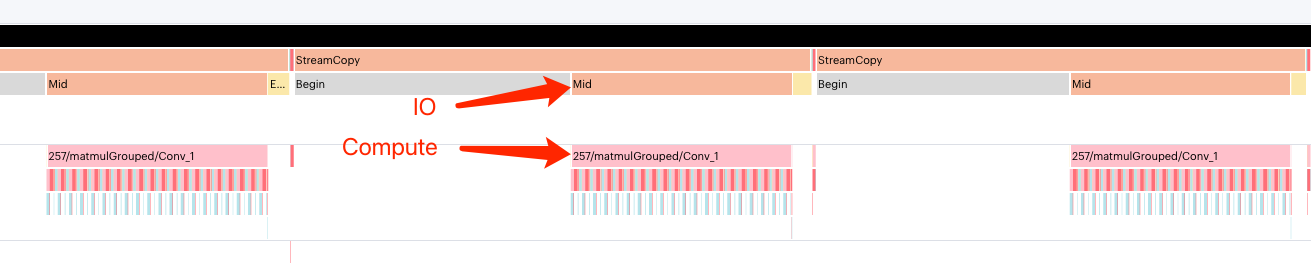# 5.3. Overlap I/O

The IPU execution of an inference model is generally divided into three stages:

1. Load: Copy input data from the host to the IPU

2. Compute: Model computing

3. Store: Copy result data from the IPU to the host

These three stages are executed serially, which means that the computing resources of the IPU are idle while data is transferred in the Load and Store stages. In some models, with large input and output data, the performance of the whole model is limited by I/O. For this kind of model, enabling overlap I/O can overlap the computing stage and the I/O stage. This improves the utilisation rate of the computing resources of the IPU.

## 5.3.1. Principle

The principle of overlap I/O is to divide all the tiles on the IPU into two groups, namely compute tiles and I/O tiles. Compute tiles perform all computation, while I/O tiles only handle transferring data with the host. In this way, the stages of Load, Compute and Store form a three-level pipeline in a computational flow, which overlaps compute and I/O to improve the utilisation rate of the computing resources of the IPU.

## 5.3.2. Configuring I/O tiles

To enable overlap I/O, you only need to set one parameter, the number of I/O tiles. The number of I/O tiles can be adjusted to optimise the throughput of the transmission. To calculate the number of I/O tiles, you can divide the sum of the tensor sizes of all input and output by the SRAM size available for each tile and then round to the next power of 2.

• Configuring I/O tiles with the PopRT CLI `--num_io_tiles` parameter:

```poprt \
--input_model model.onnx \
--export_popef \
--output_dir model \
--num_io_tiles 128
```
```opts = poprt.compiler.CompilerOptions()
opts.num_io_tiles = 128
```

## 5.3.3. Debugging

You can use the PopVision Graph Analyser to display the overlap between I/O and Compute stages to determine whether overlap I/O would improve your throughput. Fig. 5.3 shows an example of the output of the PopVision Graph Analyser.Fig. 5.3 PopVision Graph Analyser shows overlap between I/O and Compute

## 5.3.4. Concurrent requests

Since the three stages of inference form a three-stage pipeline through overlap I/O, sufficient concurrent data must be fed to the IPU in order to keep the pipeline full. At least three threads are required to feed the concurrent data to the IPU through the multi-threading mode.

## 5.3.5. Example

The following is a simple example using overlap I/O:

Listing 5.1 simple_overlapio.py
```  1# Copyright (c) 2022 Graphcore Ltd. All rights reserved.
2import argparse
4
5import numpy as np
6import onnx
7
8from onnx import helper
9
10from poprt import runtime
11from poprt.compiler import Compiler, CompilerOptions
12from poprt.runtime import RuntimeConfig
13
14'''
15PopRT use OverlapInnerLoop strategy as default exchange strategy.
16There are two loops in the main program: outer loop and inner loop.
17Each batch data needs to be processed in three pipeline stages: load/compute/store.
18Therefore, in order to enable the pipeline to run normally, at least three threads
19are required to feed data to the pipeline at the same time.
20==============================================================
21OverlapInnerLoop:
22- Boxes denote subgraphs / subgraph Ops / loops
23- Inputs/outputs are loop carried in order
24
25.- outer loop ----------------------------------------.
26|                  .- inner loop -.                   |
27| load - compute - | - store      |                   |
28|           load - | - compute -- | - store           |
29|                  |   load ----- | - compute - store |
30|                  '--------------'                   |
31'-----------------------------------------------------'
32         ^^^^^^^       ^^^^^^^        ^^^^^^^
33         overlap       overlap        overlap
34
35==============================================================
36'''
37
38
39def compile(model: onnx.ModelProto, args):
40    """Compile ONNX to PopEF."""
41    model_bytes = model.SerializeToString()
42    outputs = [o.name for o in model.graph.output]
43
44    options = CompilerOptions()
45    options.batches_per_step = args.batches_per_step
46    options.num_io_tiles = args.num_io_tiles
47
48    executable = Compiler.compile(model_bytes, outputs, options)
49    return executable
50
51
52def run(executable, args):
53    """Run PopEF."""
54    # Create model runner
55    config = RuntimeConfig()
56    config.timeout_ns = 0
57    # Create model runner
58    model_runner = runtime.Runner(executable, config)
59
60    inputs_info = model_runner.get_execute_inputs()
61    outputs_info = model_runner.get_execute_outputs()
62
63    # Run in multiple threads
64    def execute(bps, inputs_info, outputs_info):
65        inputs = {}
66        outputs = {}
67
68        for input in inputs_info:
69            inputs[input.name] = np.random.uniform(0, 1, input.shape).astype(
70                input.numpy_data_type()
71            )
72        for output in outputs_info:
73            outputs[output.name] = np.zeros(
74                output.shape, dtype=output.numpy_data_type()
75            )
76
77        # To correctly generate the popvision report, iteration must be a
78        # multiple of batches_per_step and greater than 2 * batches_per_step
79        # There are 3 threads, so the total number feed into IPU is 3 * iteration
80        iteration = bps
81        for _ in range(iteration):
82            model_runner.execute(inputs, outputs)
83
90                target=execute, args=(args.batches_per_step, inputs_info, outputs_info)
91            )
92        )
93
95        t.start()
96
98        t.join()
99    print(f"Complete.")
100
101
102def default_model():
103    TensorProto = onnx.TensorProto
104
105    nodes = []
106    num_matmuls = 4
107    nodes.append(helper.make_node("Expand", ["input", "shape"], ["Act0"]))
108    for i in range(num_matmuls):
109        nodes.append(helper.make_node("MatMul", [f"Act{i}", "Weight"], [f"Act{i+1}"]))
110    nodes.append(
111        helper.make_node("ReduceMean", [f"Act{num_matmuls}"], ["output"], axes=[0, 1])
112    )
113
114    graph = helper.make_graph(
115        nodes,
116        "matmul_test",
117        [
118            helper.make_tensor_value_info("input", TensorProto.FLOAT, (256, 256)),
119        ],
120        [helper.make_tensor_value_info("output", TensorProto.FLOAT, (256, 256))],
121        [
122            helper.make_tensor(
123                "shape",
124                TensorProto.INT64,
125                ,
126                np.array([4, 4, 256, 256], dtype=np.int64),
127            ),
128            helper.make_tensor(
129                "Weight",
130                TensorProto.FLOAT,
131                (4, 4, 256, 256),
132                np.random.randn(4, 4, 256, 256),
133            ),
134        ],
135    )
136    opset_imports = [helper.make_opsetid("", 11)]
137    original_model = helper.make_model(graph, opset_imports=opset_imports)
138    return original_model
139
140
141if __name__ == '__main__':
142    parser = argparse.ArgumentParser(
143        description='Convert onnx model and run it on IPU.'
144    )
146        '--batches_per_step',
147        type=int,
148        default=16,
149        help="The number of on-chip loop count.",
150    )
`Download simple_overlapio.py`